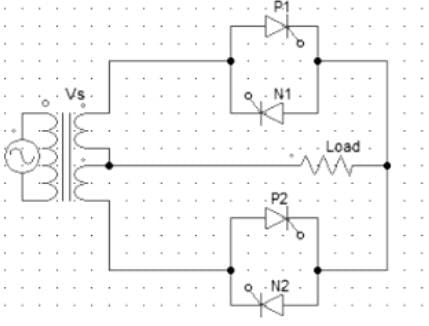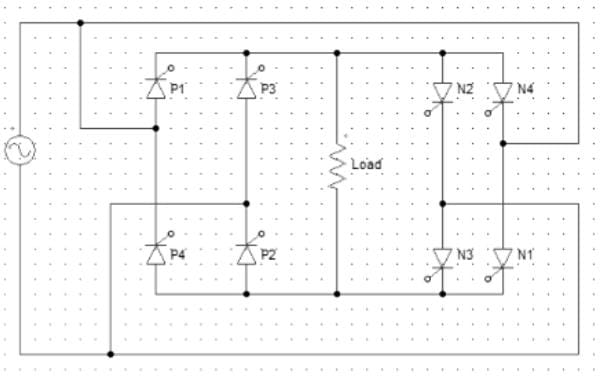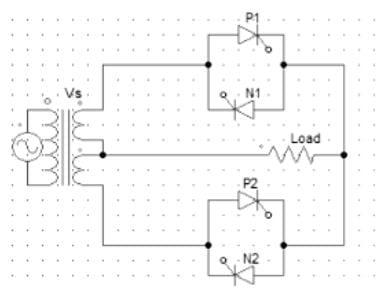Test: Cyclo Converters

# Test: Cyclo Converters

Test Description

## 10 Questions MCQ Test GATE Electrical Engineering (EE) 2023 Mock Test Series | Test: Cyclo Converters

Test: Cyclo Converters for Electrical Engineering (EE) 2023 is part of GATE Electrical Engineering (EE) 2023 Mock Test Series preparation. The Test: Cyclo Converters questions and answers have been prepared according to the Electrical Engineering (EE) exam syllabus.The Test: Cyclo Converters MCQs are made for Electrical Engineering (EE) 2023 Exam. Find important definitions, questions, notes, meanings, examples, exercises, MCQs and online tests for Test: Cyclo Converters below.
Solutions of Test: Cyclo Converters questions in English are available as part of our GATE Electrical Engineering (EE) 2023 Mock Test Series for Electrical Engineering (EE) & Test: Cyclo Converters solutions in Hindi for GATE Electrical Engineering (EE) 2023 Mock Test Series course. Download more important topics, notes, lectures and mock test series for Electrical Engineering (EE) Exam by signing up for free. Attempt Test: Cyclo Converters | 10 questions in 30 minutes | Mock test for Electrical Engineering (EE) preparation | Free important questions MCQ to study GATE Electrical Engineering (EE) 2023 Mock Test Series for Electrical Engineering (EE) Exam | Download free PDF with solutions
 1 Crore+ students have signed up on EduRev. Have you?
Test: Cyclo Converters - Question 1

### Applications of cycloconverters include

Detailed Solution for Test: Cyclo Converters - Question 1

Speed of induction machines can be controlled by controlling the input frequency. In induction heating, eddy current is proportional to the square of input frequency.

Test: Cyclo Converters - Question 2

### The single phase bridge type cycloconverter uses __________ number of SCRs.

Detailed Solution for Test: Cyclo Converters - Question 2

4 negative and 4 positive SCRs are employed in bridge type cycloconverter.

Test: Cyclo Converters - Question 3

### The principle of three phase cycloconverter is to

Detailed Solution for Test: Cyclo Converters - Question 3

The basic principle of three phase cycloconverter is to vary progressively the firing angle of the controlling devices.

Test: Cyclo Converters - Question 4

In a three phase half-wave cycloconverter ___________

Detailed Solution for Test: Cyclo Converters - Question 4

During the conversion process, the current flows in both the directions, hence both inverting and converting action takes place.

Test: Cyclo Converters - Question 5

A cycloconverter is a _________

Detailed Solution for Test: Cyclo Converters - Question 5

A cycloconverter converters input power at one frequency to output power at a different frequency with one-stage conversion.

Test: Cyclo Converters - Question 6

The single phase mid-point type cycloconverter uses __________ number of SCRs.

Detailed Solution for Test: Cyclo Converters - Question 6

2 negative and 2 positive SCRs are employed in mid-point type cycloconverter.

Test: Cyclo Converters - Question 7

In the positive half cycle from ωt = 0 to πDetailed Solution for Test: Cyclo Converters - Question 7

In the positive half cycle, upper terminal is positive of the upper secondary and the lower terminal is negative for the lower secondary. Hence, P1 and N2 are forward biased.

Test: Cyclo Converters - Question 8

In the positive half cycle, _________ SCRs are forward biased.Detailed Solution for Test: Cyclo Converters - Question 8

Pairs P1, P2 and N1, N2 are forward biased from ωt = 0 to π.

Test: Cyclo Converters - Question 9

In three phase cycloconverters, the reduction factor is given by

Detailed Solution for Test: Cyclo Converters - Question 9

Reduction factor = output frequency/input frequency.

Test: Cyclo Converters - Question 10

In the below given cycloconverter circuit, _________ and _________ conduct in one cycle together.Detailed Solution for Test: Cyclo Converters - Question 10

In the positive half cycle, upper terminal is positive of the upper secondary and the lower terminal is negative for the lower secondary. Hence, P1 and N2 are forward biased. And likewise, N1 and P2 will conduct in the next half cycle.

## GATE Electrical Engineering (EE) 2023 Mock Test Series

21 docs|273 tests
 Use Code STAYHOME200 and get INR 200 additional OFF Use Coupon Code
Information about Test: Cyclo Converters Page
In this test you can find the Exam questions for Test: Cyclo Converters solved & explained in the simplest way possible. Besides giving Questions and answers for Test: Cyclo Converters, EduRev gives you an ample number of Online tests for practice

## GATE Electrical Engineering (EE) 2023 Mock Test Series

21 docs|273 tests